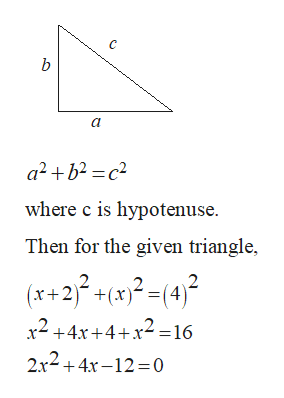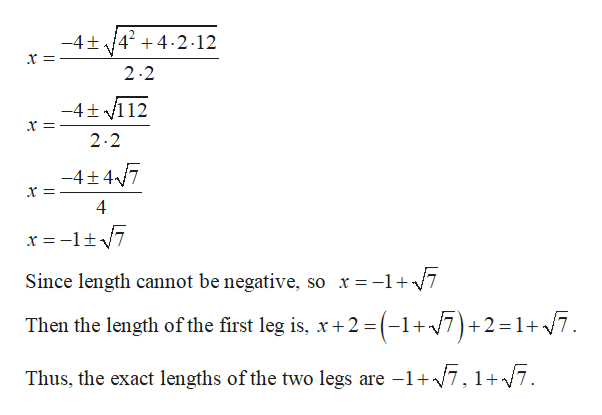# The first leg of a right triangle is 2 cm longer than its second leg. If the hypotenuse has a length of 4 cm, find the exact lengths of the two legs.

Question

The first leg of a right triangle is 2 cm longer than its second leg. If the hypotenuse has a length of 4 cm, find the exact lengths of the two legs.

check_circleExpert Solution
Step 1

Let the length of the second leg be x.

Then the length of the first leg is x+2.

From the given, the length of the hypotenuse is 4 cm.

By Pythagorean Theorem, for a right triangle,help_outlineImage TranscriptioncloseC b a2+b2-c2 where c is hypotenuse Then for the given triangle, (x+2)+(x)2 4)2 x2+4x+4+x2=16 2x2+4x-12=0 fullscreen
Step 2help_outlineImage Transcriptionclose-4t424.2-12 2.2 -4t 112 2.2 -4+ 47 Since length cannot be negative, so r= -1+7 Then the length of the first leg is, x+2 (-1+7) +2=17 Thus, the exact lengths of the two legs are -1+7,1+/7. fullscreen

### Want to see the full answer?

See Solution

#### Want to see this answer and more?

Solutions are written by subject experts who are available 24/7. Questions are typically answered within 1 hour*

See Solution
*Response times may vary by subject and question
Tagged in

### Algebra